home | O'Reilly's CD bookshelfs | FreeBSD | Linux | Cisco | Cisco Exam

#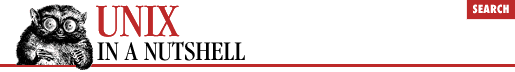Chapter 12Nroff and Troff## 12.9 Special Characters

This subsection lists the following special characters:

• Characters that reside on the standard fonts

• Miscellaneous characters

• Bracket building symbols

• Mathematics symbols

• Greek characters

The characters in the first table below are available on the standard fonts. The characters in the remaining tables are available only on the special font.

Table 12.1: On the Standard Fonts
Char Input Character Name
' ' Close quote
` ` Open quote
- \(em 3\4 em dash
- - Hyphen or
- \(hy Hyphen
- \- Minus in current font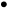\(bu Bullet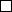\(sq Square
_ \(ru Rule
¼ \(14 1/4
½ \(12 1/2
¾ \(34 3/4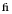\(fi fi ligature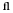\(fl fl ligature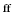\(ff ff ligature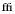\(Fi ffi ligature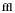\(Fl ffl ligature
° \(de Degree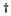\(dg Dagger
' \(fm Foot mark
¢ \(ct Cent sign
® \(rg Registered
Table 12.2: Miscellaneous Characters
Char Input Character Name
§ \(sc Section
´ \(aa Acute accent
` \(ga Grave accent
_ \(ul Underrule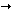\(-> Right arrow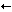\(<- Left arrow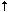\(ua Up arrow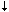\(da Down arrow
| \(br Box rule\(dd Double dagger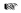\(rh Right hand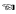\(lh Left hand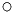\(ci Circle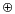\(vs Visible space indicator (ditroff only)
Table 12.3: Bracket Building Symbols
Char Input Character Name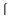\(lt Left top of big curly bracket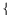\(lk Left center of big curly bracket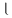\(lb Left bottom of big curly bracket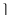\(rt Right top of big curly bracket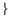\(rk Right center of big curly bracket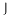\(rb Right bottom of big curly bracket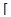\(lc Left ceiling (left top) of big square bracket
| \(bv Bold vertical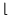\(lf Left floor left bottom of big square bracket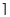\(rc Right ceiling (right top) of big square bracket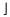\(rf Right floor (right bottom) of big square bracket
Table 12.4: Mathematics Symbols
Char Input Character Name
+ \(pl Math plus
- \(mi Math minus
= \(eq Math equals
* \(** Math star
/ \(sl Slash (matching backslash)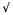\(sr Square root
\(rn Root en extender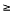\(>= Greater than or equal to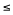\(<= Less than or equal to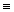\(== Identically equal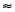\(~~ Approx equal
~ \(ap Approximates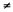\(!= Not equal
× \(mu Multiply
÷ \(di Divide
± \(+- Plus-minus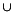\(cu Cup (union)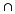\(ca Cap (intersection)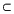\(sb Subset of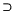\(sp Superset of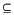\(ib Improper subset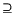\(ip Improper superset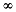\(if Infinity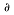\(pd Partial derivative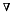\(gr Gradient
¬ \(no Not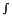\(is Integral sign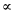\(pt Proportional to\(es Empty set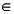\(mo Member of
| \(or Or

### 12.9.1 Greek Characters

Characters that have equivalents as uppercase English letters are available on the standard fonts; otherwise, the characters below exist only on the special font.

Char Input Char Name Char Input Char Name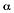\(*a alpha A \(*A ALPHA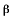\(*b beta B \(*B BETA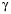\(*g gamma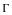\(*G GAMMA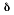\(*d delta\(*D DELTA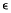\(*e epsilon E \(*E EPSILON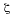\(*z zeta Z \(*Z ZETA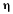\(*y eta H \(*Y ETA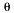\(*h theta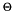\(*H THETA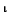\(*i iota I \(*I IOTA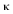\(*k kappa K \(*K KAPPA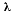\(*l lambda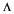\(*L LAMBDA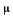\(*m mu M \(*M MU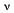\(*n nu N \(*N NU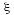\(*c xi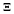\(*C XI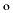\(*o omicron O \(*O OMICRON\(*p pi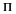\(*P PI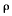\(*r rho P \(*R RHO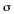\(*s sigma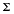\(*S SIGMA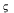\(ts terminal sigma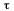\(*t tau T \(*T TAU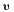\(*u upsilon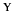\(*U UPSILON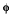\(*f phi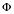\(*F PHI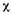\(*x chi X \(*X CHI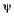\(*q psi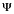\(*Q PSI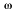\(*w omega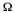\(*W OMEGA12.8 Predefined Number Registers13. mm Macros